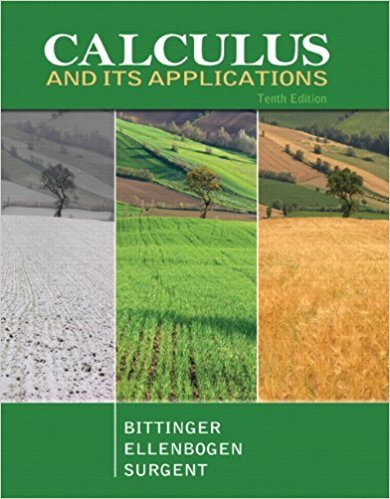×
×

# Solutions for Chapter 2.6: Marginals and Differentials## Full solutions for Calculus and Its Applications | 10th Edition

ISBN: 9780321694331Solutions for Chapter 2.6: Marginals and Differentials

Solutions for Chapter 2.6
4 5 0 409 Reviews
10
5
##### ISBN: 9780321694331

This textbook survival guide was created for the textbook: Calculus and Its Applications, edition: 10. This expansive textbook survival guide covers the following chapters and their solutions. Calculus and Its Applications was written by and is associated to the ISBN: 9780321694331. Since 69 problems in chapter 2.6: Marginals and Differentials have been answered, more than 18160 students have viewed full step-by-step solutions from this chapter. Chapter 2.6: Marginals and Differentials includes 69 full step-by-step solutions.

Key Calculus Terms and definitions covered in this textbook
• Absolute value of a complex number

The absolute value of the complex number z = a + b is given by ?a2+b2; also, the length of the segment from the origin to z in the complex plane.

• Bounded interval

An interval that has finite length (does not extend to ? or -?)

• Continuous function

A function that is continuous on its entire domain

• Equivalent equations (inequalities)

Equations (inequalities) that have the same solutions.

• Event

A subset of a sample space.

• Feasible points

Points that satisfy the constraints in a linear programming problem.

• Geometric series

A series whose terms form a geometric sequence.

• Order of an m x n matrix

The order of an m x n matrix is m x n.

• Ordered pair

A pair of real numbers (x, y), p. 12.

• Placebo

In an experimental study, an inactive treatment that is equivalent to the active treatment in every respect except for the factor about which an inference is to be made. Subjects in a blind experiment do not know if they have been given the active treatment or the placebo.

• Probability function

A function P that assigns a real number to each outcome O in a sample space satisfying: 0 … P1O2 … 1, P12 = 0, and the sum of the probabilities of all outcomes is 1.

• Quartic function

A degree 4 polynomial function.

• Reference triangle

For an angle ? in standard position, a reference triangle is a triangle formed by the terminal side of angle ?, the x-axis, and a perpendicular dropped from a point on the terminal side to the x-axis. The angle in a reference triangle at the origin is the reference angle

• Reflexive property of equality

a = a

• Riemann sum

A sum where the interval is divided into n subintervals of equal length and is in the ith subinterval.

• Sine

The function y = sin x.

• Sum identity

An identity involving a trigonometric function of u + v

A graph in which (-x, y) is on the graph whenever (x, y) is; or a graph in which (-r, -?) or (r, ?, -?) is on the graph whenever (r, ?) is

• Union of two sets A and B

The set of all elements that belong to A or B or both.

• Vertical translation

A shift of a graph up or down.

×